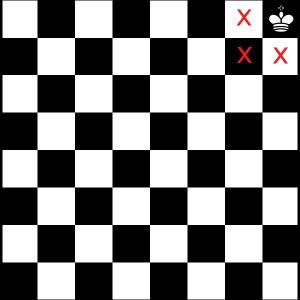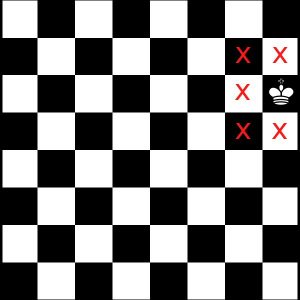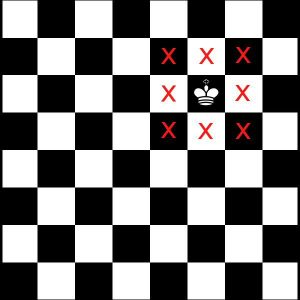# Counting Kings

#### (A new problem of the week)

Having discussed counting earlier this week, let’s take a look at a different kind of counting. The subject of combinatorics (the study of counting) arises in many guises: probability, sets, geometry. Here, we look at a relatively basic type of problem that involves the same sort of organized thinking that we used to count vertices.

Here is the problem, from a couple months ago:

Q)  How many ways are there to place two kings on a chessboard so that they are safe from each other?

I tried this question starting that if the king is at corner blocks then there would be total 12 places where the other king cannot be.  Then in corner rows I am not able to find as there are overlappings. Please help me how to calculate in the corner rows. Please send the solution till the end so that I don’t have problems latest in this question.

Our first issue in answering this question is to agree on terminology (what is a “corner block” and a “corner row”?); then we need to determine how the student is looking at the problem itself. Doctor Rick replied:

“I tried this question starting that if the king is at corner blocks then there would be total 12 places where the other king cannot be.”

Let’s start by understanding this part. I think I see where you got 12: is it the total number of places where the other king cannot be, for all four corner positions of the first king? If so, that’s not what matters here. Rather, take the number of places where the second king cannot be, if the first king is in any one of the corner positions. Use that to find the number of places where the second king can be in each case, and multiply that by the number of corner positions (4). This will give you the total number of “safe” placements of two kings for which the first king is in a corner square.

In approaching a counting question, we first have to be sure what is being asked; often, questions are asked from a perspective unfamiliar to students new to the topic. Here, the question is about “ways to place two kings”, which might be restated as “pairs of squares on the board such that kings in them are not attacking one another”. The concept of counting pairs, rather than individual items, is not something we do every day! So it is understandable that this student at first thinks of counting all squares where any king might be, rather than taking them separately for each place where the first could be.

So far, we have four places for the first king to be (the corners), each of which excludes four squares for the other (where the first king is, and three squares attacking it):Doctor Rick continued:

“Then in corner rows I am not able to find as there are overlappings. Please help me how to calculate in the corner rows.”

I am not sure what you mean by “corner rows”. What I would be thinking about next is the non-corner edge squares — that is, squares that are on one of the four edges but not in one of the corners. How many such squares are there, on a standard 8×8 chess board?

I also don’t know what you mean by overlappings. Can you explain that? Again, what we care about is the number of safe positions for the second king if the first king is in any one of these non-corner edge squares.

My guess is that the student is talking about places along the edges other than the corners (as Doctor Rick is), and is thinking that there are two many of these squares to count all at once as he has been trying to do. If so, then the advice to emphasize the word “each” may be all he needs.

There are 6 non-corner edge squares on each side, for a total of 24 such squares; for each of these, there are 6 squares where the second king can’t be:The student then wrote twice, ten minutes apart, with updates on his thinking. First:

Ok, I got that I had to calculate the number of places where other king can be.

So if king A is at the corner then king B is anywhere in the rest (16 – 4)×4=36 places.  Then there are 6 places in corner edges (taking last two edges) where king A can be. For any of King A’s position there will be (6×4)=24 places left for king B. Now in the middle there are 24 places left where king A can be.  For each of king A’s position there are 24 – 9=15 places left for king B. Have I done correct till here?  What should I do next?

It appears that the student is either thinking of only a quarter of the board at a time (16 squares), or is still thinking of all four corners being occupied at once rather than one at a time. I can’t quite see where many of these numbers come from, though.

But then he wrote again:

If the white king is in a corner (4 possibilities), the black king can be at any of 64 − 4 positions.

If the white king is at the boundary, but not in a corner (24 possibilities), the black king can be at any of 64 − 6 positions.

In all other cases for the white king (36 possibilities), the black king can be at any of 64 − 9 positions.

In total: 4⋅60 + 24⋅58 + 36⋅55.

Is this correct now?

This is looking good. Sometimes just explaining your thinking to someone else (or to yourself!) can help you see where it is wrong, so you can correct yourself without additional input. We have now included interior squares for the first king, which each exclude 9 for the second:“If the white king is in a corner (4 possibilities), the black king can be at any of 64 − 4 positions.”

Correct: king B can’t be in the same corner or any of the three positions adjacent to it.

“If the white king is at the boundary, but not in a corner (24 possibilities), the black king can be at any of 64 − 6 positions”

Correct: there are 24 non-corner boundary positions, and six positions for king B are ruled out in each case.

“In all other cases for the white king (36 possibilities), the black king can be at any of 64 − 9 positions.”

Correct again.

“In total: 4⋅60 + 24⋅58 + 36⋅55.

Is this correct now.”

There is only one more thing we need to consider. The problem asked, “How many ways are there to place two kings on a chessboard so that they are safe from each other?” It isn’t clear whether the two kings are to be considered distinguishable or not. I would guess, importing basic knowledge of chess into the problem, that the two kings are of different colors (one white and one black). In that case, the configuration with the white king in the upper left corner and the black king two places to its right, for example, is different from the configuration with the black king in the upper left corner and the white king two places to its right. If so, you’ve got it, I believe. But if the kings are indistinguishable, we need to divide your result by 2 to account for each pair of configurations counting as a single “way to place two kings”.

It is important in counting different ways to do something, to consider not only what the “something” is that we are to do, but also what counts as “different”. It seems more or less clear what would be intended here, but another interpretation is conceivable.

So our final answer is that there are 240 ways to place the kings if the white king is in a corner; 1392 ways to place them if the white king is along an edge; and 1980 ways if the white king is in the interior, for a total of 3612 ways. And if all that mattered was which squares they are on, not which king is where, then we would divide that by 2 (since we would have counted each arrangement twice) to get 1806.

What did we have to do in order to carry out this count? We didn’t list all the ways, which would have been very difficult; instead, we used a “divide and conquer” strategy, breaking the possibilities into three groups based on how many squares are around each king; and we used multiplication to handle the “for each” relationship. Within each category, we used multiplication and subtraction to find a total excluding some. But in order to carry all this out, we first had to clearly understand the problem — and clearly communicate our own meanings to one another. Counting may sound like baby stuff, but it involves many skills that are useful throughout mathematics.

## There’s nothing new for the Math Doctors!

Now, I have found that almost any question we get, something close to it will have been discussed here before! After writing the above, I decided to check, and I found this question from 15 years ago:

Chess King Positions

On a regular 8x8 board with 64 squares, the total possible positions is 3,612. If the board is then transformed to a 13x9 with 117 squares, how does one go about figuring this out?

I have tried simple cross multiplication but that cannot be correct.

So this student had seen our problem and knew the answer, but wanted help solving a larger version with a rectangular board. Doctor Jeremiah answered, first showing the work for the already-known problem:

The two kings cannot be in squares right beside each other (because one of them would be in check) so for each position of the first king, the second king cannot be in every remaining square.

If the first king is in a corner, then the other king cannot be on that square or the three surrounding it. For an 8x8 board that means the number of possibilities for the second king is 60 squares when the first king is in one of the four corners.

If the first king is on a side, then the other king cannot be on that square or the five surrounding it. For an 8x8 board that means the number of possibilities for the second king is 58 squares when the first king is on one of the 24 side squares.

If the first king is in the center somewhere, then the other kingcannot be on that square or the eight surrounding it. For an 8x8 board that means the number of possibilities for the second king is 55 squares when the first king is on one of the 36 inner squares.

Total possibilities = 60 squares for each of four corners plus 58 squares for each of 24 side squares plus 55 squares for each of 36 inner squares: 4x60+24x58+36x55 = 3612 possibilities

Now, how would you do a 13x9 board?

John had an answer, presumably having been inspired by a clear presentation of the solution:

The answer should be 12,764 positions on a 13x9 board. Corner 113x4 = 452; Sides 111x36 = 3996; and Center 108x77 = 8316.

Doctor Jeremiah confirmed that result, showing the details of the various sub-counts:

If the first king is in a corner, then the other king cannot be on that square or the three surrounding it.  For a 13x9 board that means the number of possibilities for the second king is (13x9-4) squares when the first king is in one of the four corners.  For all four corners the total combinations = 4 x (13x9-4) = 452.

If the first king is on a side, then the other king cannot be on that square or the five surrounding it.  For a 13x9 board that means the number of possibilities for the second king is (13x9-6) squares when the first king is on one of the side squares.  The total number of side squares is (13-2)+(13-2)+(9-2)+(9-2) = 36.  For all the side squares the total combinations = 36 x (13x9-6) = 3996.

If the first king is in the center somewhere, then the other king cannot be on that square or the eight surrounding it. For a 13x9 board that means the number of possibilities for the second king is (13x9-9) squares when the first king is on one of the inner squares.  The total number of inner squares is the board size minus the side squares minus the corners of 13x9 - 36 - 4 = 77.  For all the inner squares the total combinations = 77 x (13x9-9) = 8316.

Total possibilities = (13x9-4) squares for each of four corners plus (13x9-6) squares for each of 36 side squares plus (13x9-8) squares for each of 77 inner squares:

4 x (13x9-4) + 36 x (13x9-6) + 77 x (13x9-9) = 452+3996+8316

Which is exactly what you got!

This site uses Akismet to reduce spam. Learn how your comment data is processed.